##### CHAPTERS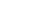# Choosing the Best Machine Learning Classification Model and Avoiding Overfitting

Modeling with machine learning is a challenging but valuable skill for anyone working with data. No matter what you use machine learning for, chances are you have encountered questions around classification and overfitting along the way. This ebook shows you how to mitigate the effects of these challenges using MATLAB®.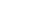##### CHAPTERS

Classification Models

# Classification Models

## Choosing the Right Classification Model

#### What is a data classification model?

Classification models are used to assign items to a discrete group or class based on a specific set of features.

#### Why is it so hard to get right?

Each model has its own strengths and weaknesses in a given scenario. There is no cut-and-dried flowchart that can be used to determine which model you should use without grossly oversimplifying the considerations. Choosing a data classification model is also closely tied to the business case and a solid understanding of what you are trying to accomplish.

#### What can you do to choose the right model?

To begin with, make sure you can answer the following questions:

• How much data do you have and is it continuous?
• What type of data is it?
• What are you trying to accomplish?
• How important is it to visualize the process?
• How much detail do you need?
• Is storage a limiting factor?Follow the Heart Sound Classifier example

When you’re confident you understand the type of data you’re going to be working with and what it will be used for, you can start looking at the strengths of various models. There are some generic rules of thumb to help you choose the best classification model, but these are just starting points. If you are working with a large amount of data (where a small variance in performance or accuracy can have a large effect), then choosing the right approach often requires trial and error to achieve the right balance of complexity, performance, and accuracy. The following sections describe some of the common models that are useful to know.

#### Classification Cross-Validation

Cross-validation is a model assessment technique used to evaluate a machine learning algorithm's performance when making predictions on new datasets it has not been trained on. This is done by partitioning a dataset and using a subset to train the algorithm and the remaining data for testing. This technique is discussed in more detail in Chapter 3.

## Naïve BayesIf the data is not complex and your task is relatively simple, try a Naïve Bayes algorithm. It’s a high-bias/low-variance classifier, which has advantages over logistic regression and nearest neighbor algorithms when working with a limited amount of data available to train a model.

Naïve Bayes is also a good choice when CPU and memory resources are a limiting factor. Because Naïve Bayes is very simple, it doesn’t tend to overfit data, and can be trained very quickly. It also does well with continuous new data used to update the classifier.

If the data grows in size and variance and you need a more complex model, other classifiers will probably work better. Also, its simple analysis is not a good basis for complex hypotheses.

Naïve Bayes is often the first algorithm scientists try when working with text (think spam filters and sentiment analysis). It’s a good idea to try this algorithm before ruling it out.

## k-Nearest Neighbor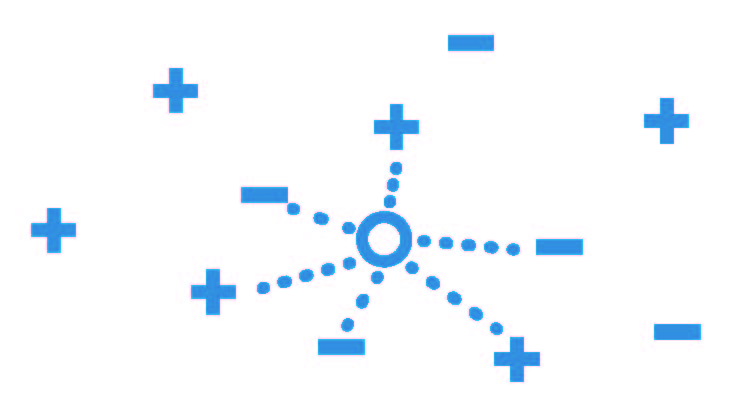Categorizing data points based on their distance to other points in a training dataset can be a simple yet effective way of classifying data. k-nearest neighbor (kNN) is the “guilty by association” algorithm.

kNN is an instance-based lazy learner, which means there’s no real training phase. You load the training data into the model and let it sit until you actually want to start using the classifier. When you have a new query instance, the kNN model looks for the specified k number of nearest neighbors; so if k is 5, then you find the class of 5 nearest neighbors. If you are looking to apply a label or class, the model takes a vote to see where it should be classed. If you’re performing a regression problem and want to find a continuous number, take the mean of f values of k nearest neighbors.

Although the training time of kNN is short, actual query time (and storage space) might be longer than that of other models. This is especially true as the number of data points grows because you’re keeping all the training data, not just an algorithm.

The greatest drawback to this method is that it can be fooled by irrelevant attributes that obscure important attributes. Other models such as decision trees are better able to ignore these distractions. There are ways to correct for this issue, such as applying weights to your data, so you’ll need to use your judgment when deciding which model to use.

## Decision Trees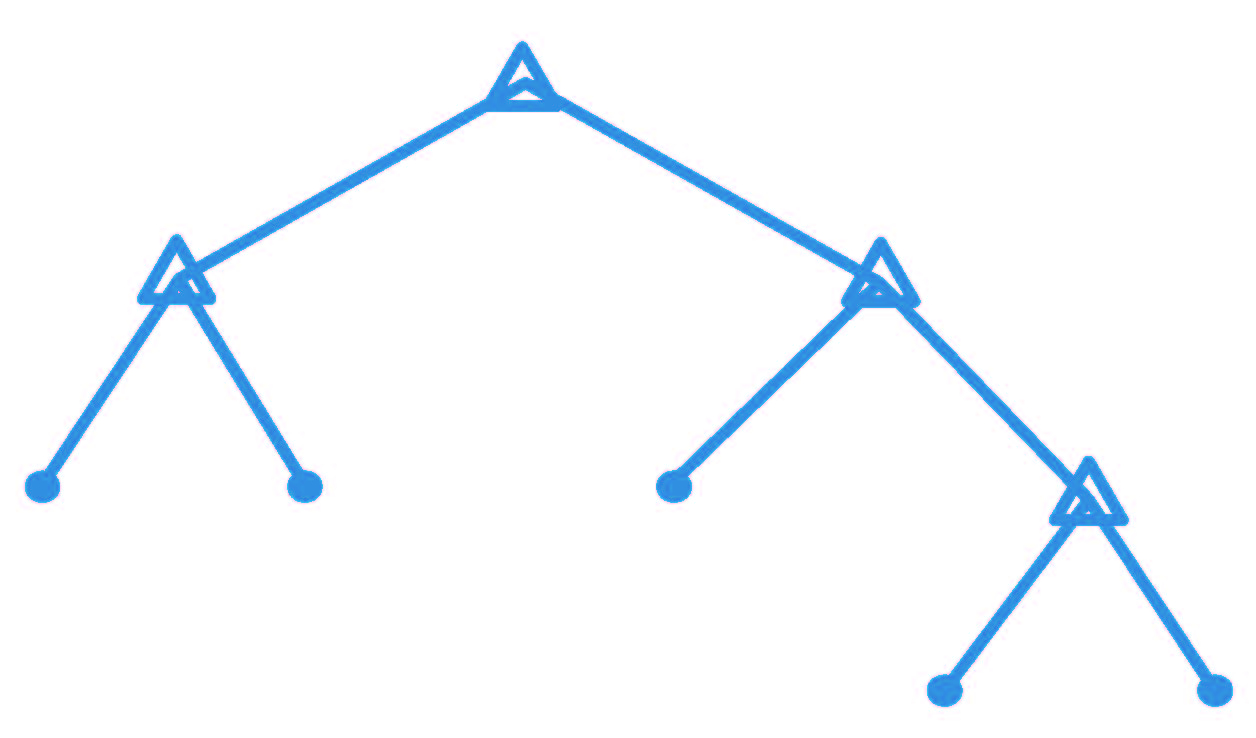To see how a decision tree predicts a response, follow the decisions in the tree from the root (beginning) node down to a leaf node which contains the response. Classification trees give responses that are nominal, such as true or false. Regression trees give numeric responses.

Decision trees are relatively quick and easy to follow; you can see a full representation of the path taken from root to leaf. This is especially useful if you need to share the results with people interested in how a conclusion was reached.

The main disadvantage of decision trees is that they tend to overfit, but there are ensemble methods to counteract this. Toshi Takeuchi has written a good example (for a Kaggle competition) that uses a
bagged decision tree
to determine how likely someone would be to survive the Titanic disaster.

## Support Vector Machine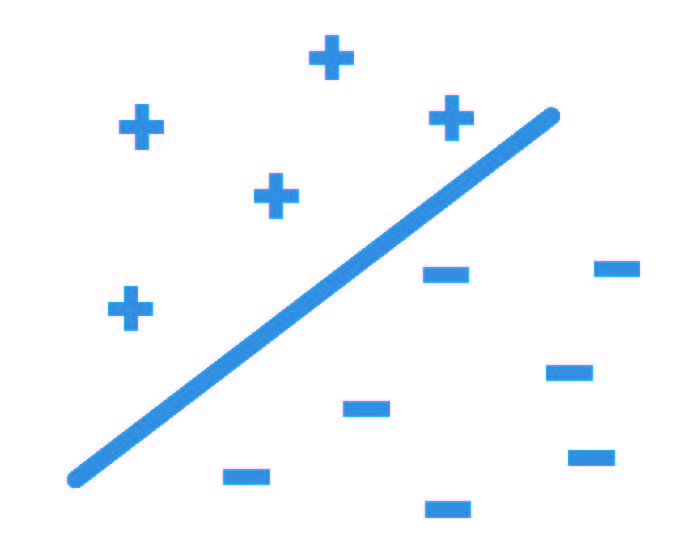You might use a support vector machine (SVM) when your data has exactly two classes. An SVM classifies data by finding the best hyperplane that separates all data points of one class from those of the other class (the best hyperplane for an SVM is the one with the largest margin between the two classes). You can use an SVM with more than two classes, in which case the model will create a set of binary classification subproblems (with one SVM learner for each subproblem).

There are a couple of strong advantages of using an SVM. First, it is extremely accurate and tends not to overfit data. Second, linear support vector machines are relatively easy to interpret. Because SVM models are very fast, once your model has been trained you can discard the training data if you have limited memory available. It also tends to handle complex, nonlinear classification very well by utilizing a technique called the “kernel trick.”

However, SVMs need to be trained and tuned up front, so you need to invest time in the model before you can begin to use it. Also, its speed is heavily impacted if you are using the model with more than two classes.

## Neural Networks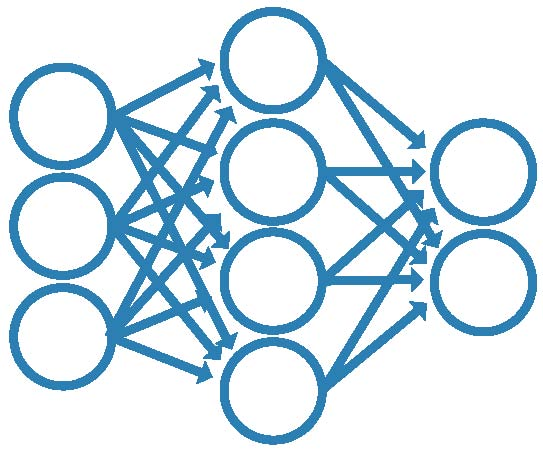An artificial neural network (ANN) can learn, and therefore be trained to find solutions, recognize patterns, classify data, and forecast future events. People often use ANNs to solve more complex problems, such as character recognition, stock market prediction, and image compression.

The behavior of a neural network is defined by the way its individual computing elements are connected and by the strengths of those connections, or weights. The weights are automatically adjusted by training the network according to a specified learning rule until it performs the desired task correctly.

For experienced users, ANNs are great at modeling nonlinear data with a high number of input features. When used correctly, ANNs can solve problems that are too difficult to address with a straightforward algorithm. However, neural networks are computationally expensive, it is difficult to understand how an ANN has reached a solution (and therefore infer an algorithm), and fine-tuning an ANN is often not practical—all you can do is change the inputs of your training setup and retrain.

#### The greatest drawback to kNNs is that they can be fooled by irrelevant attributes that obscure important attributes. Applying weights to your data can correct for this issue.

See more characteristics of classifiers
##### CHAPTERS

Classification Models

See more characteristics of classifiers